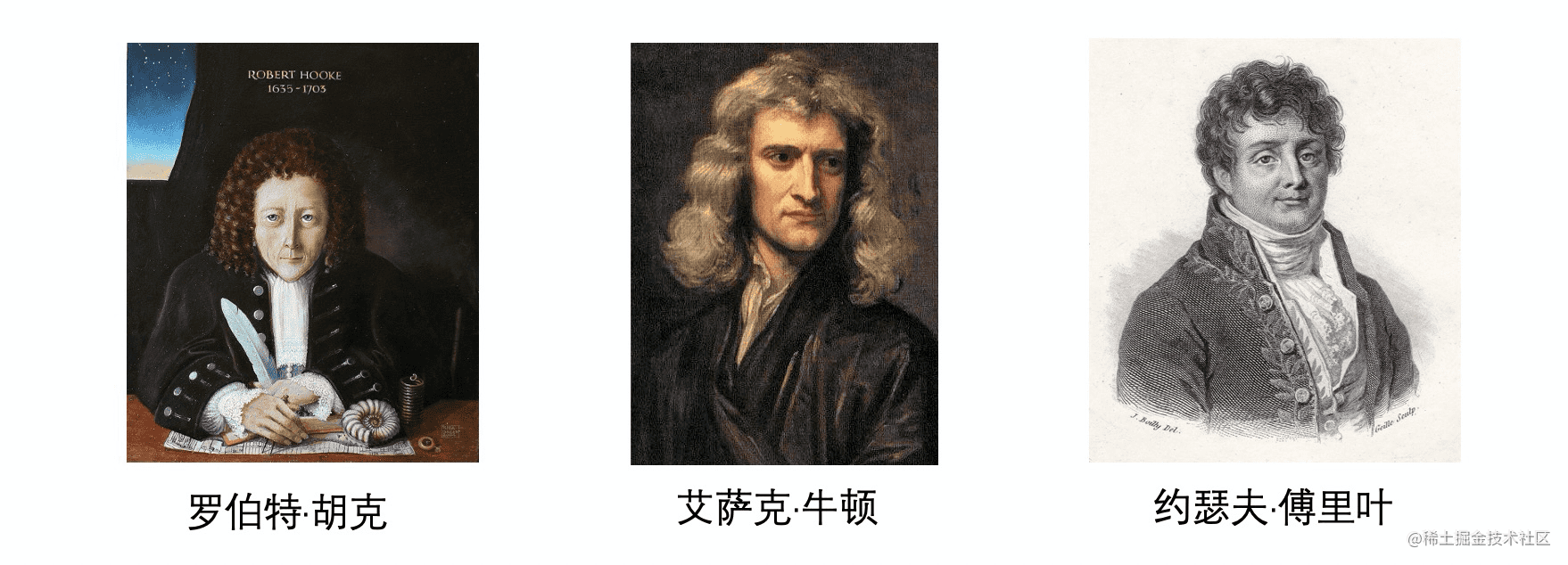前端弹性动画与 framer-motion 动画库初探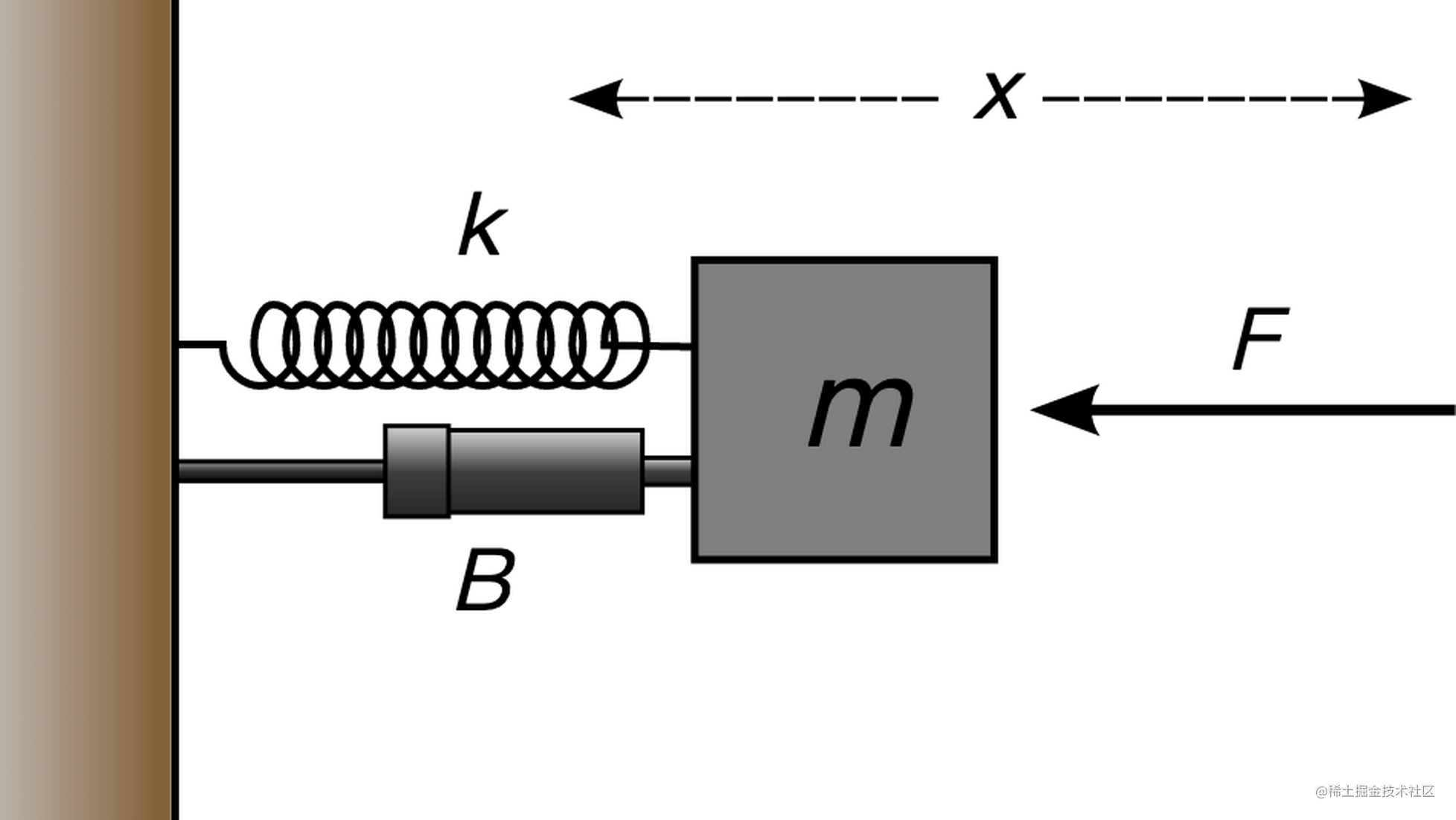动画效果的真实体验

timing-function 的不足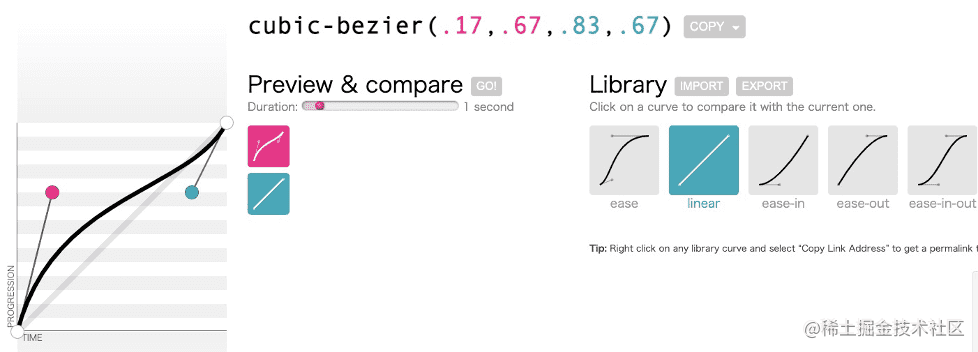弹簧-阻尼系统中的运动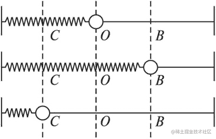$F_s=-kX$

$k$ 为弹簧劲度系数

$F_d=-cv$

$F=F_s+F_d=-kX-cv$

$F=ma$

$\begin{cases} F=-kX-cv \\ F=ma \end{cases}$

$a=-kX-cv$

$X$代表物体从其平衡位置开始移动。这就意味着，如果我们想从 $x=x$$x=1$，那么我们要每次移动 $x-1$  才能到达那个指定位置（微分的思想）。

$X=x-1$

$a=-k(x-1)-cv$

$x=f(t)$

$v=\frac{dx}{dt}=f'(t)$

$a=\frac{dv}{dt}=f''(t)$

$\begin{cases} a=-k(x-1)-cv \\ \\ a=\dfrac{dv}{dt}=f''(t) \\ \\ v=\dfrac{dx}{dt}=f'(t) \\ \\ x=f(t) \end{cases}$

$f''(t)=-k(f(t)-1)-cf'(t)$

$f(0)=0$
$f'(0)=0$

$\begin{cases} f(0) = 0 \\ f'(0) = 0 \\ f''(t) = -180(f(t) - 1) - 12f'(t) \end{cases}$

f(0) = 0; f'(0) = 0; f''(t) = -180(f(t) - 1) - 12f'(t)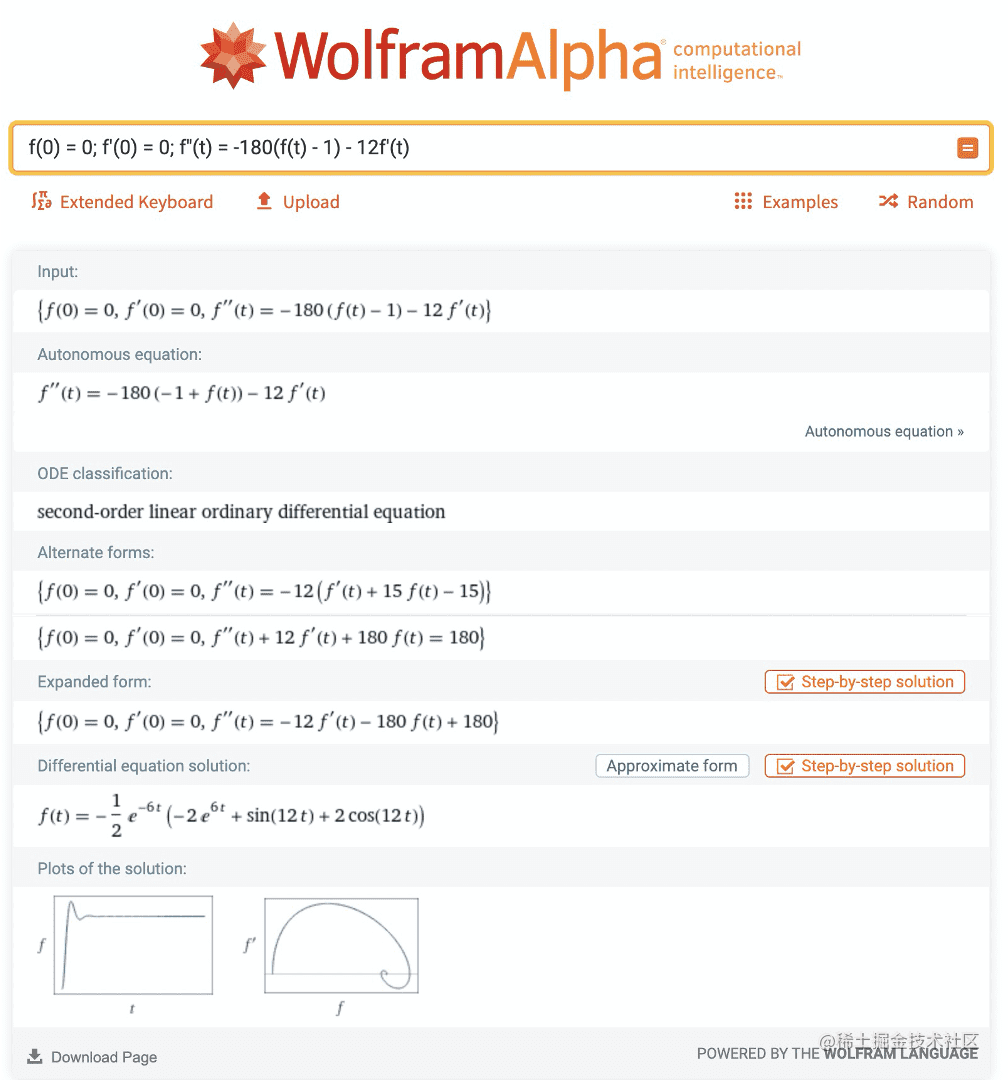$f(t)=-\frac{1}{2}e^{-6t}(-2e^{6t}+sin(12t)+2cos(12t))$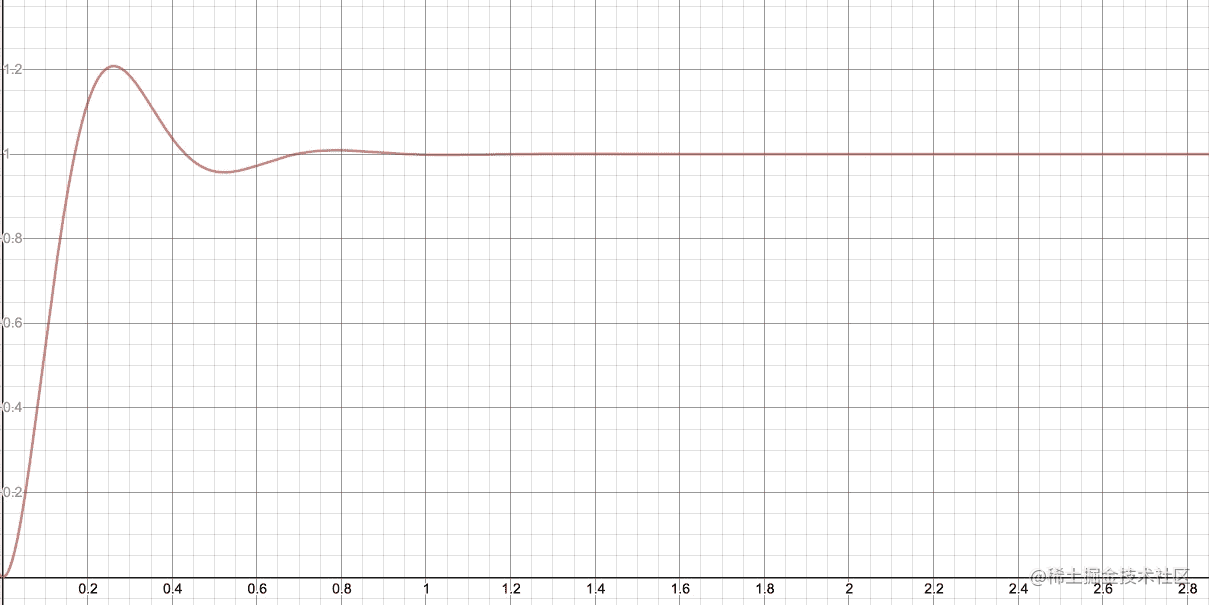简谐运动

• 零阻尼 (zero damping)：图中虚线为零阻尼的情况，物体将永不停歇的做周期运动
• 欠阻尼 (light damping)：图中蓝线为欠阻尼情况，物体在平衡位置震荡，但振幅减小，最终回到平衡位置
• 临界阻尼 (critical damping)：图中红线为临界阻尼情况，物体以最快速度回到平衡位置。现实生活中，许多大楼内房间或卫生间的门上在装备自动关门的扭转弹簧的同时，都相应地装有阻尼铰链，使得门的阻尼接近临界阻尼，这样人们关门或门被风吹动时就不会造成太大的声响，同时又能以最快速度关闭。另外汽车的避震弹簧也是使用了临界阻尼的原理
• 过阻尼 (over damping)：图中绿线为过阻尼情况，物体以非常缓和的方式回到平衡位置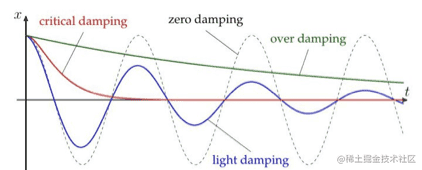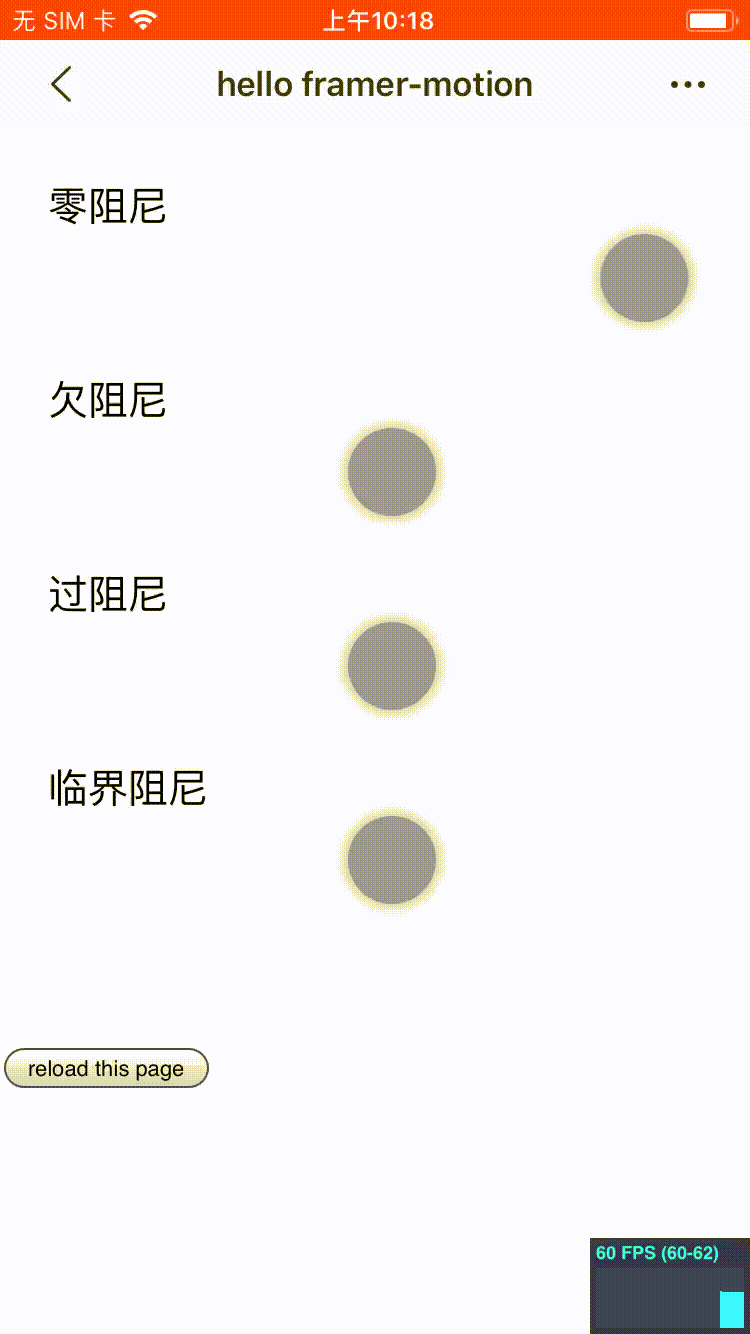点击或扫码体验基于 React 的弹性动画库

• react-motion
• spring 动画的提出者，不支持 hooks api，3年内未更新
• react-spring
• 灵感来源于 react-motion，支持 hooks，功能强大
• framer-motion
• 支持 hooks，功能强大，同时增加了很多声明式属性，api 简洁友好

react-motion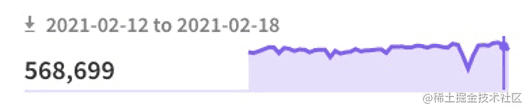react-spring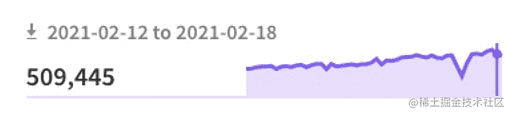framer-motion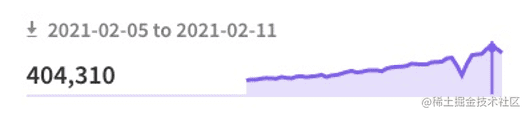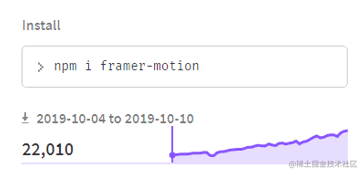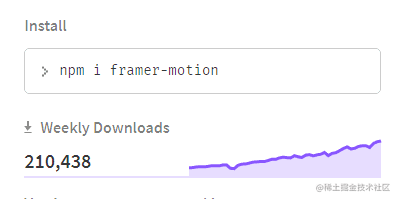2020年10月的周下载量几乎是2019年同期的10倍，这大部分得益于其 api 的友好性。接下来将一起探索一下 framer-motion 的使用方式。

framer-motion

A production-ready motion library for React. Utilize the power behind Framer, the best prototyping tool for teams. Proudly open source.

framer motion 是一个生产级的 React 动画库，为他们自家原型工具产品 Framer 提供了支持，并自豪的进行了开源。事实上，framer motion 作为动画库，提供了一些极其简洁的 api 帮助我们创建复杂的动效，这些 api 帮助然我们抽象出动画背后的复杂性，让创建动画变得简单。

• 声明式api
• 组件间共享布局动画
• 手势支持

弹簧阻尼 demo

import React from 'react'
import { motion } from 'framer-motion'

import './index.css'

function index() {
return (
<>
<div className="wrap">
<div className="title">零阻尼</div>
<motion.div
className="ball"
animate={{
x: 150,
transition: {
type: 'spring',
damping: 0,
},
}}
/>
</div>
<div className="wrap">
<div className="title">欠阻尼</div>
<motion.div
className="ball"
animate={{
x: 150,
transition: {
type: 'spring',
damping: 2,
},
}}
/>
</div>
<div className="wrap">
<div className="title">过阻尼</div>
<motion.div
className="ball"
animate={{
x: 150,
transition: {
type: 'spring',
damping: 100,
},
}}
/>
</div>
<div className="wrap">
<div className="title">临界阻尼</div>
<motion.div
className="ball"
animate={{
x: 150,
transition: {
type: 'spring',
damping: 17,
},
}}
/>
</div>

<br />
<br />
<br />
<button
type="button"
onClick={() => {
}}
>
</button>
</>
)
}

export default index

demo 页面链接 gaohaoyang.github.io/framer-moti…

• motion 为前缀的 HTML 或 SVG 标签结合在一起创建的基础组件
• 通过 prop 与组件对接的 api

animate={{
x: 150,
transition: {
type: 'spring', // 弹簧动画
damping: 0, // 阻尼值
},
}}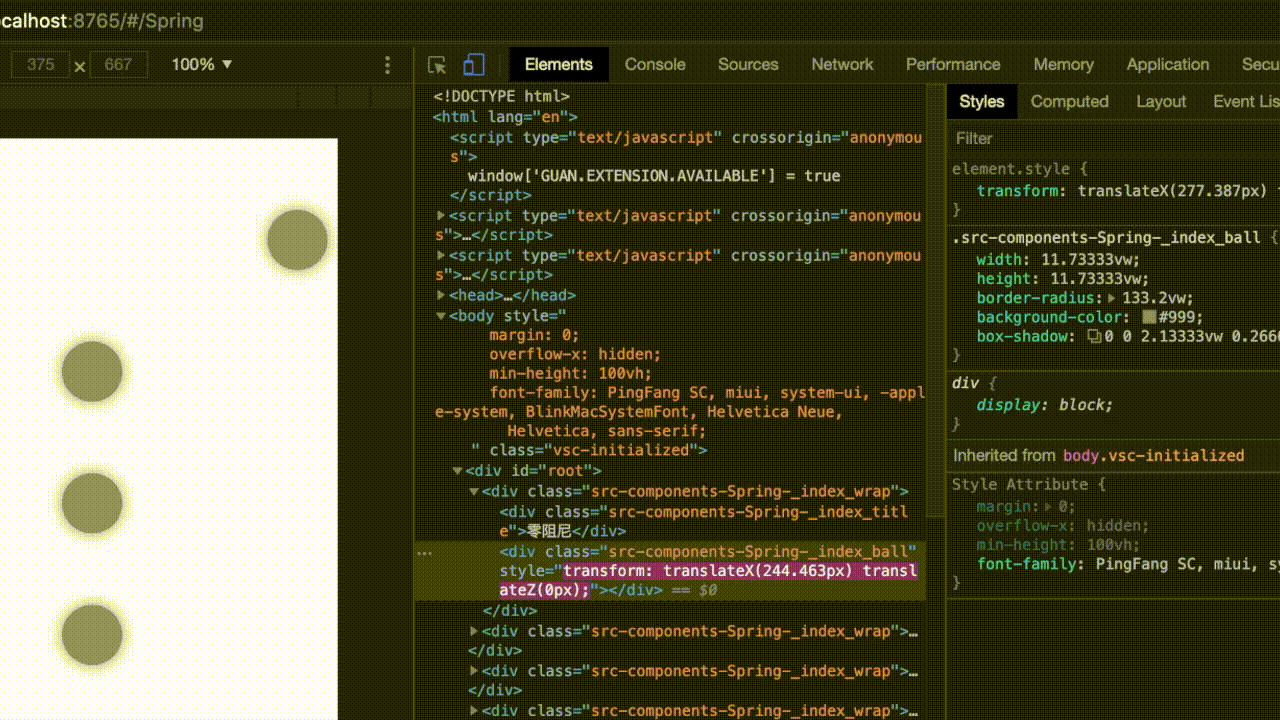import React, { useState } from 'react'
import { motion, AnimatePresence } from 'framer-motion'

function index() {
const [toggleShow, setToggleShow] = useState(true)
return (
<>
<br />
<br />
<button
onClick={() => {
setToggleShow((pre) => !pre)
}}
type="button"
>
toggleShow
</button>
<br />
<br />

<AnimatePresence>
{toggleShow && (
<motion.div
style={{
backgroundColor: '#ddd',
width: '50vw',
height: '80vw',
}}
key="modal"
initial={{ opacity: 0 }}
animate={{ opacity: 1, x: 100 }}
exit={{ opacity: 0, x: 0 }}
>
</motion.div>
)}
</AnimatePresence>
</>
)
}

export default index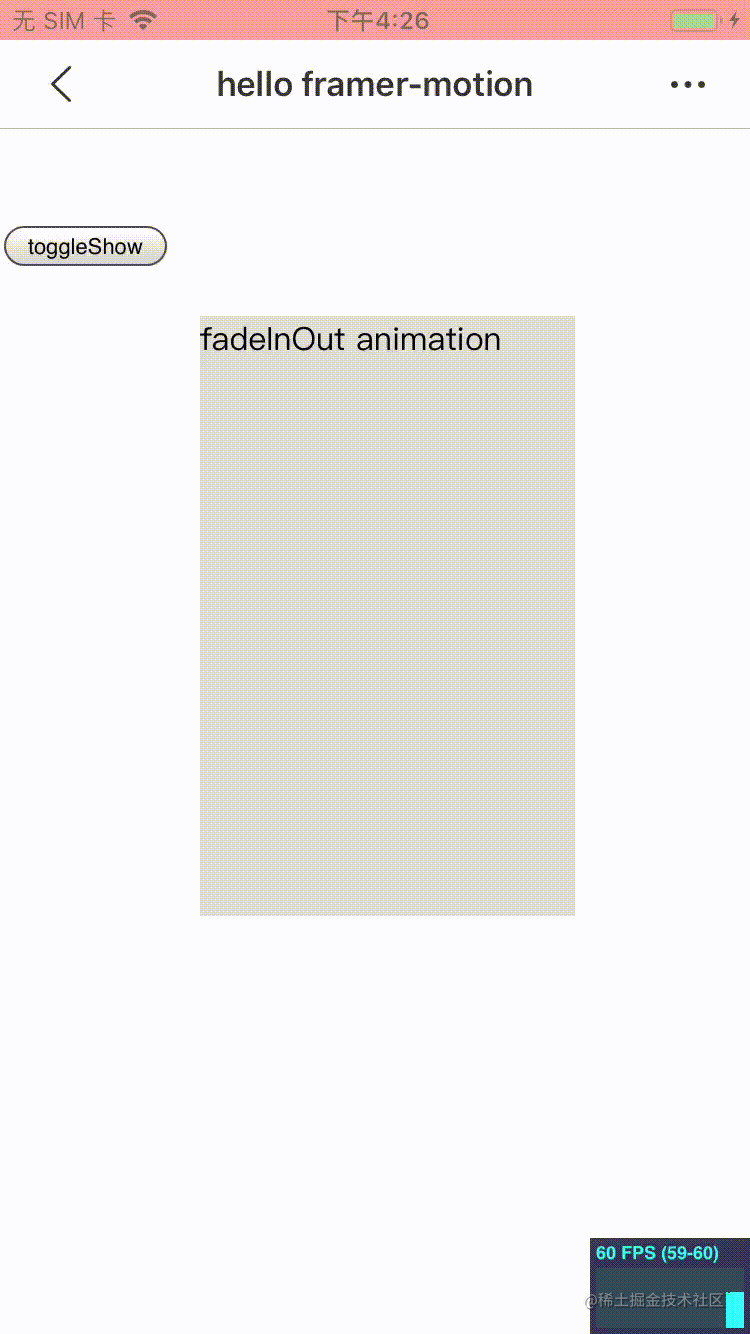点击或扫码体验exit={{ opacity: 0, x: 0 }}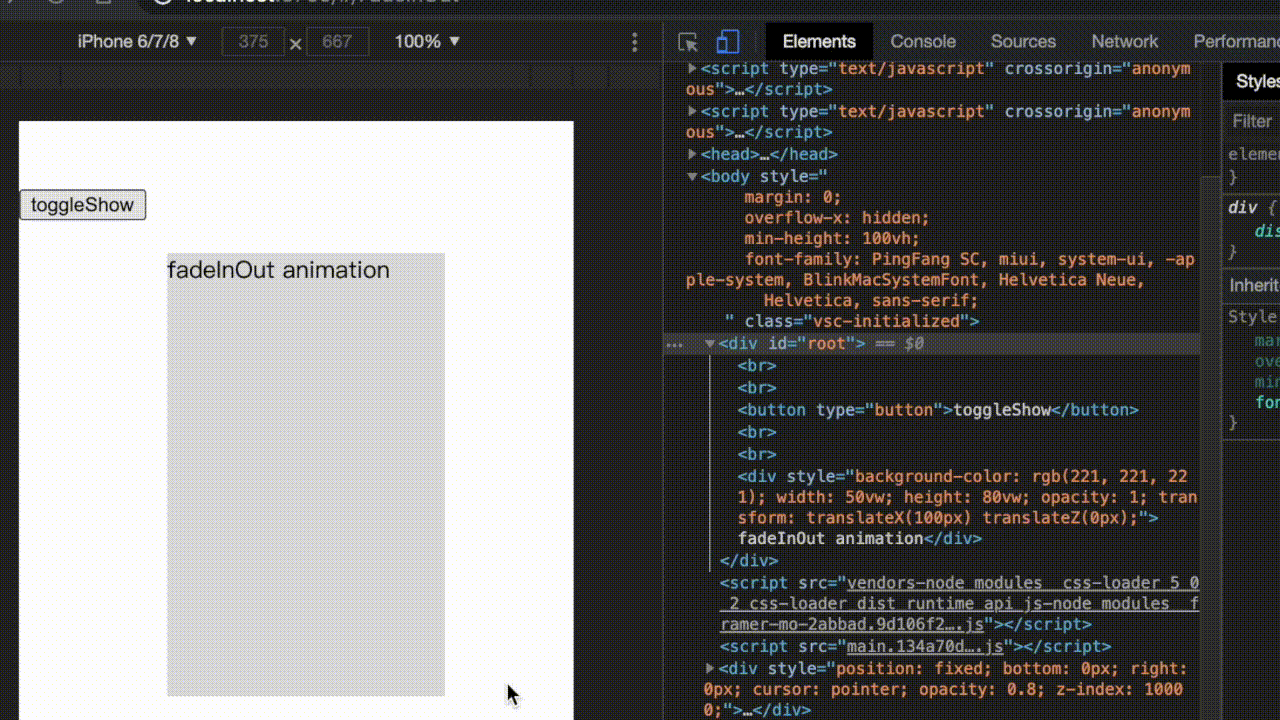drag demo

import React from 'react'
import { motion } from 'framer-motion'
import './index.css'

function index() {
return (
<div className="container">
<div
className="box"
style={{
width: '300px',
height: '500px',
}}
>
<motion.div
style={{ width: '44px', height: '44px' }}
className="ball"
drag
dragConstraints={{
top: -228,
bottom: 228,
left: -128,
right: 128,
}}
dragElastic={0.2}
/>
</div>
</div>
)
}

export default index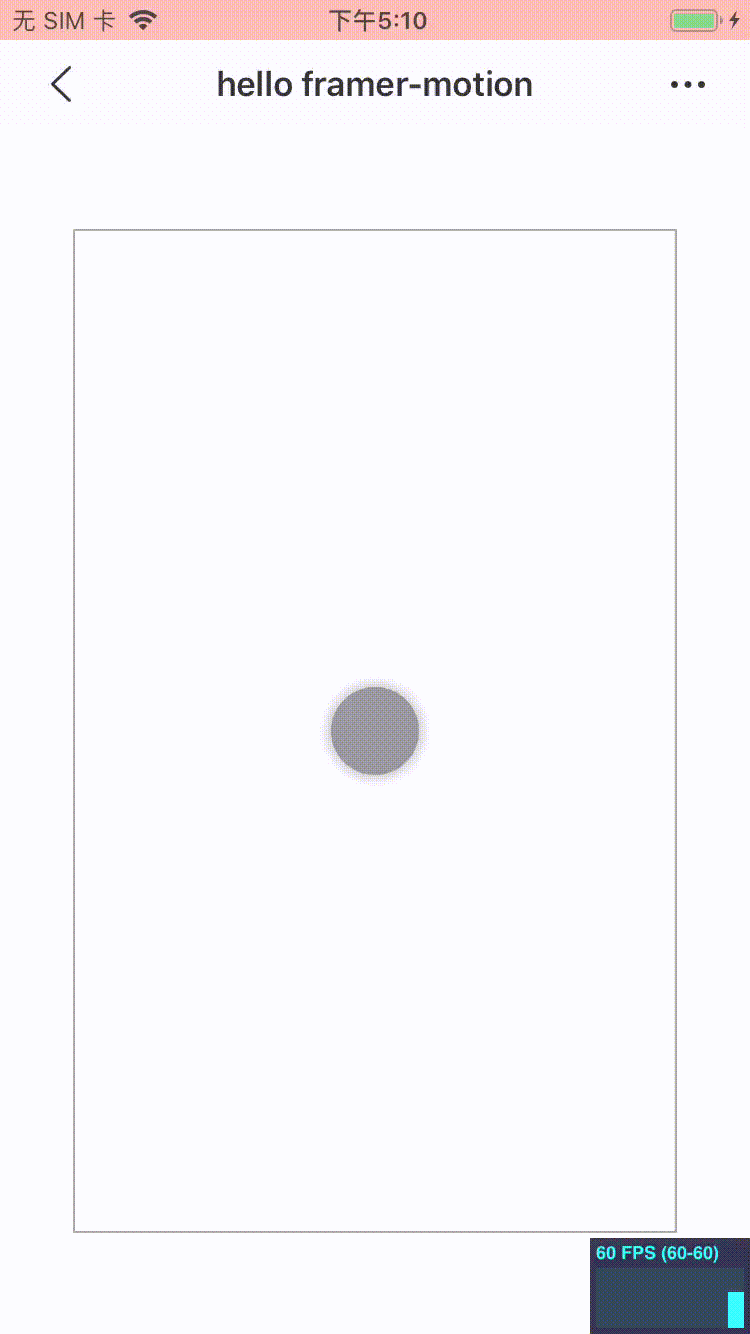点击或扫码体验transform: translate3d(128px, 228px, 0px);demo 的 git 地址：github.com/Gaohaoyang/…

framer-motion 主要 api

framer-motion 不止拥有 spring 动画，同样也具有 tween 补间动画，keyframes 动画，同时还提供了动画编排、丰富的手势、各种 hooks api，详情可以参考官网文档 www.framer.com/api/motion/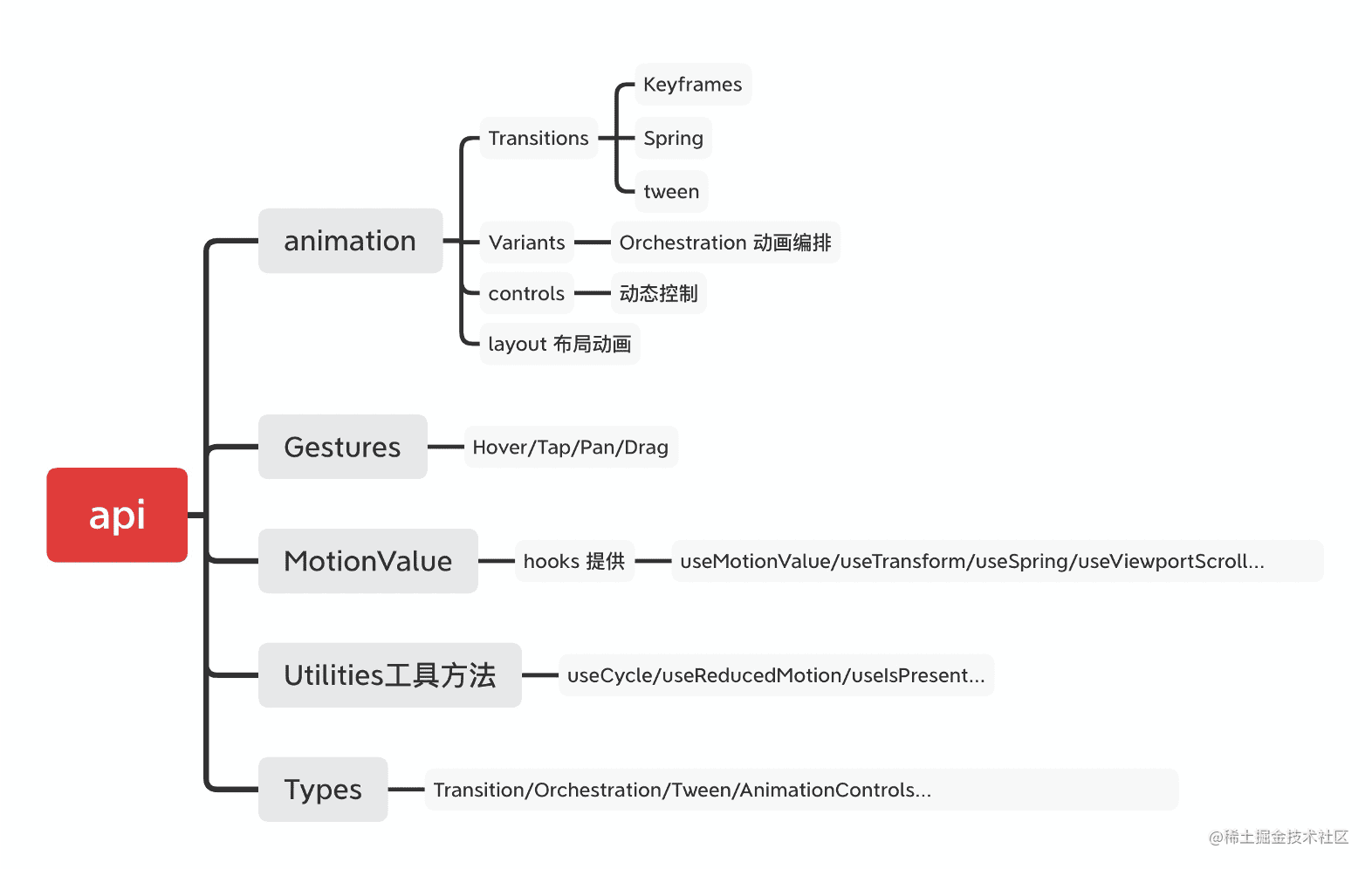总结